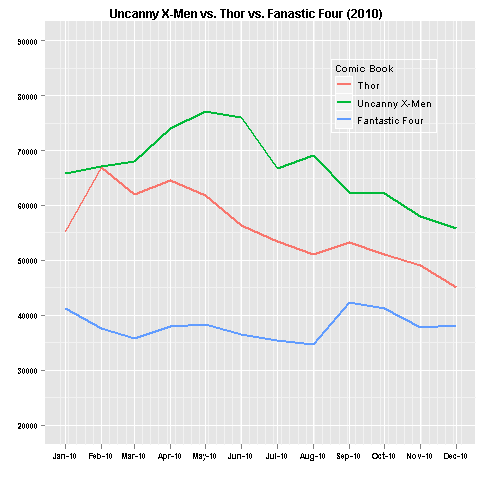Want to share your content on R-bloggers? click here if you have a blog, or here if you don't.

Three of Marvel’s longest running comic book series’ are Thor,
Uncanny X-Men, and Fantastic Four. Using data from 2010, I compare
monthly comic book sales for each series. This data only pertains
to monthly issues and not trade paperbacks. Furthermore, the series
Amazing Spider Man was not considered because it was released twice
a month.```ggplot(mydat, aes(Time)) +
geom_line(aes(y = Sold, colour = "Thor"), size = 1) +
geom_line(aes(y = Sold.1, colour = "Uncanny X-Men"), size = 1) +
geom_line(aes(y = Sold.2, colour = "Fantastic Four"), size = 1) +
opts(title = "Uncanny X-Men vs. Thor vs. Fanastic Four (2010)") +
opts(plot.title = theme_text(size = 12, face = "bold")) +
ylim(c(20000,90000)) + xlab("") + ylab("") +
opts(axis.text.y = theme_text(family = "sans", face = "bold", size = 8)) +
opts(axis.text.x = theme_text(family = "sans", face = "bold", size = 8)) +
opts(plot.margin = unit(c(0.3, 0.3, 0.3, 0.1), "lines")) +
scale_colour_discrete('Comic Book') +
opts(legend.position = c(0.8, 0.8))```